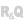HelpWLanguageWLanguage functionsControls, pages and windowsDiagram editor functionsPrefix syntaxTypes of variablesDiagram functionsSee alsoDiagram constantsDiagram functionsDiagramEndModificationDiagramGroupDiagramInfoXYDiagramLoadDiagramLoadLibraryDiagramSaveDiagramSearchDiagramSelectMinusDiagramSelectPlusDiagramShapeToImageDiagramStartModificationDiagramToImageDiagramToSVGDiagramUngroup
WINDEVWEBDEVWINDEV MobileOthersThe diagram management functions are as follows:
 DiagramEndModification Indicates the end of a series of actions performed in a Diagram document in a single event ("undo/redo"). DiagramGroup Creates a group from the elements selected in the Diagram Editor control. DiagramInfoXY Indicates the presence of a shape at a given position in the Diagram Editor control. DiagramLoad Loads a diagram into a Diagram variable from a ".wddiag" file, a memo or a binary buffer. DiagramLoadLibrary Loads a diagram file and creates a library of preset shapes from it. DiagramSave Saves a diagram as a "diag" file.New in version 28DiagramSearch Searches for shapes containing a specific text in a diagram. DiagramSelectMinus Deselects a shape in a Diagram Editor control. DiagramSelectPlus Selects a shape in a Diagram Editor control. DiagramShapeToImage Creates an image from a shape in a diagram (the shape can be displayed or not in the diagram). DiagramStartModification Indicates the start of a series of actions performed in a Diagram document in a single event ("undo/redo"). DiagramToImage Exports a diagram to a variable of type Image. DiagramToSVG Exports a diagram to an SVG image. DiagramUngroup Dissociates the different shapes of a group in a Diagram Editor control.
Multiple types of specific variables can also be used to handle diagrams: